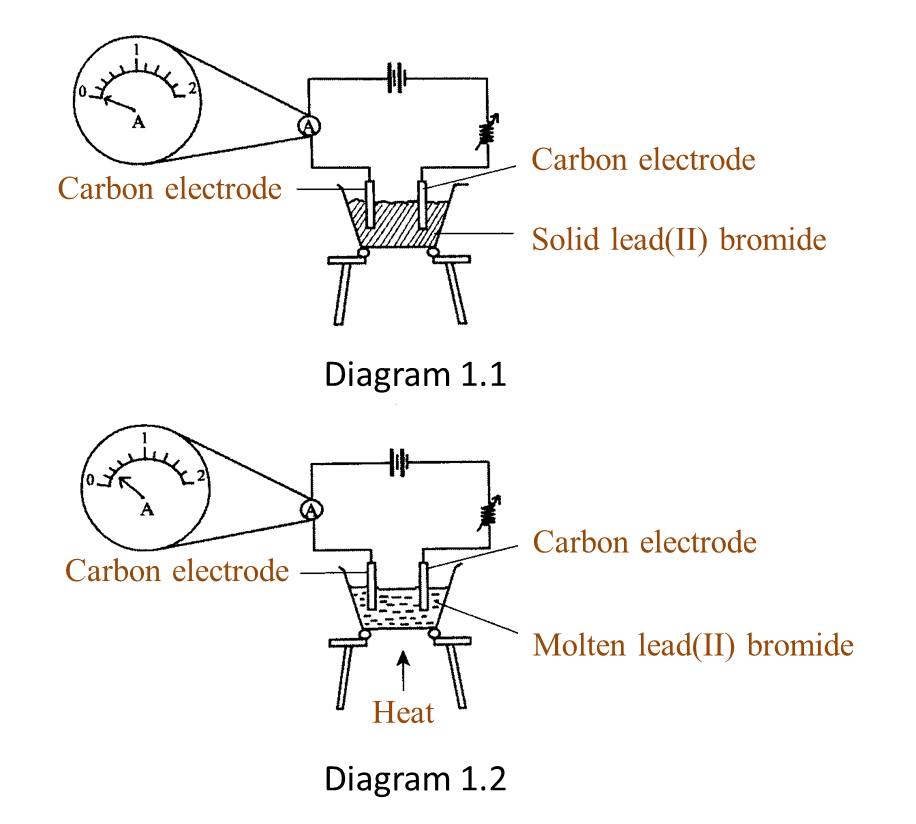Question 1:
Diagram 1.1 and Diagram 1.2 show an experiment to study the electrical conductivity of lead(II) bromide.(a)(i) Based on Diagram 1.2, what is your observation on the needle of the ammeter? [1 mark]

(ii) What is the reading of the ammeter in Diagram 1.2? [1 mark]

(b) State the variables I this experiment.
(i) Manipulated variable:
(ii) Responding variable:
[2 marks]

(c) State one inference for this experiment. [1 mark]

(d) Lead(II) bromide is an ionic compound.
State the operational definition for an ionic compound. [1 mark]

(a)(i)
The pointer of the ammeter is deflected.

(a)(ii)
0.4 A

(b)(i)
The state of the lead(II) bromide or chemical compound

(b)(ii)